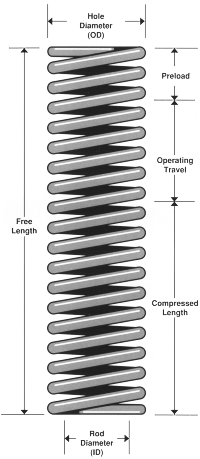# Die Spring TerminologyDie springs are characterized by the following die spring terminology.

HOLE DIAMETER This identifies the outside diameter (OD) of the die spring. Raymond die springs are available in eight different hole sizes matched to standard drill sizes. Each spring is made to fit in the hole, so the OD of the spring is actually less than the hole diameter.

ROD DIAMETER This is a nominal identification of the inside diameter (11D) of the die spring. Raymond die springs are available in eight different hole sizes matched to standard stripper bolts. Each spring is made to fit over the rod, so the ID of the spring is actually greater than the rod diameter.

FREE LENGTH The length of a die spring before it is subject to any operating force or load.

PRELOAD The distance the free length of the die spring is reduced by the pressure of the assembled tool.

OPERATING TRAVEL The distance which is subtracted from the spring length after operating force has been applied.

DEFLECTION The amount of change in spring length when force is applied.

COMPRESSED LENGTH The length of the spring after operating force has been applied. The compressed length is computed by subtracting the initial compression and the operating travel from the free length.

SOLID HEIGHT The length of a spring when it is compressed by enough load to bring all the coils into contact with each other.

REMOVE SET The manufacturing process of closing a compression spring to solid to eliminate load loss in operation.

PERMANENT SET This happens when the elastic limits are exceeded and the spring does not return to its original length when the load is released.

ELASTIC LIMIT The maximum compression stress that a die spring can endure without taking permanent set.

LOAD This is the force built up by compressing the spring. Load is expressed in terms of total pounds, which is the load on the spring per a specific unit of deflection. Load is also directly related to stress. As the spring is compressed, load is generated and stress on the coils increases.

STRESS in a spring, this describes the internal force that resists deflection under load. This force is equal to, and in the opposite direction of, the external load. Stress is expressed in thousands of pounds per square inch of sectional area.